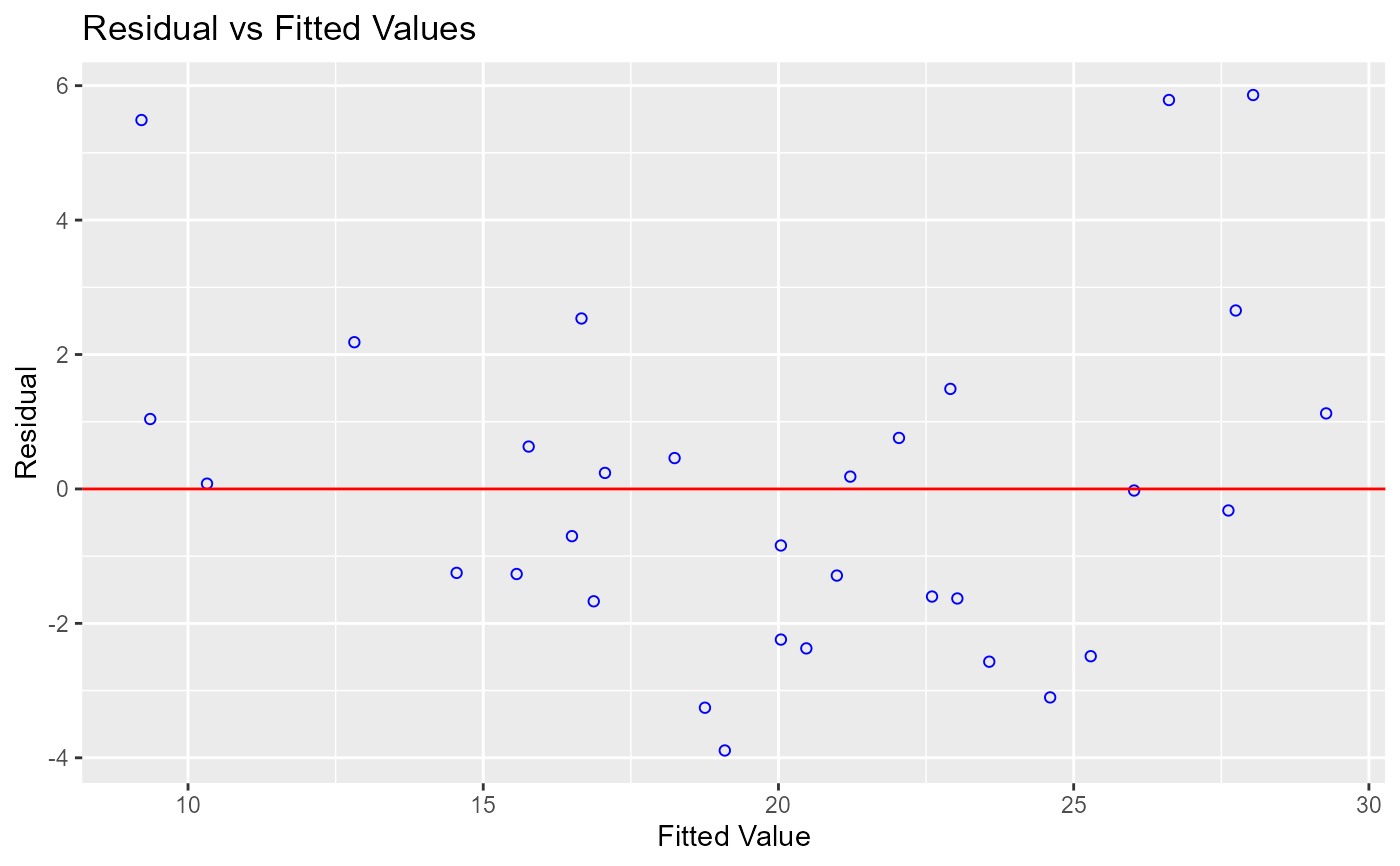Scatter plot of residuals on the y axis and fitted values on the x axis to detect non-linearity, unequal error variances, and outliers.

## Usage

ols_plot_resid_fit(model, print_plot = TRUE)

## Arguments

model

An object of class lm.

print_plot

logical; if TRUE, prints the plot else returns a plot object.

## Details

Characteristics of a well behaved residual vs fitted plot:

• The residuals spread randomly around the 0 line indicating that the relationship is linear.

• The residuals form an approximate horizontal band around the 0 line indicating homogeneity of error variance.

• No one residual is visibly away from the random pattern of the residuals indicating that there are no outliers.

Other residual diagnostics: ols_plot_resid_box(), ols_plot_resid_hist(), ols_plot_resid_qq(), ols_test_correlation(), ols_test_normality()
model <- lm(mpg ~ disp + hp + wt, data = mtcars)